Function Repository Resource:

# SqrtSpace

Move back and forth from the squared space or square root space of an algebraic number field

Contributed by: Ed Pegg Jr
 ResourceFunction["SqrtSpace"][root,pts] while tracking signs, converts Cartesian pts2 to algebraic values in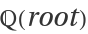or converts those values back to Cartesian.

## Examples

### Basic Examples (2)

Using ϕ, GoldenRatio or Fibonacci’s rabbit constant, convert points to the algebraic number field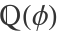and build the Fermat triangle:

 In:=Out=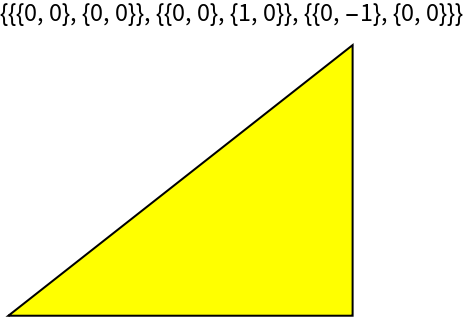Using ψ, the supergolden ratio or Narayana’s cow constant, convert points to the algebraic number field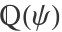and build the supergolden triangle:

 In:=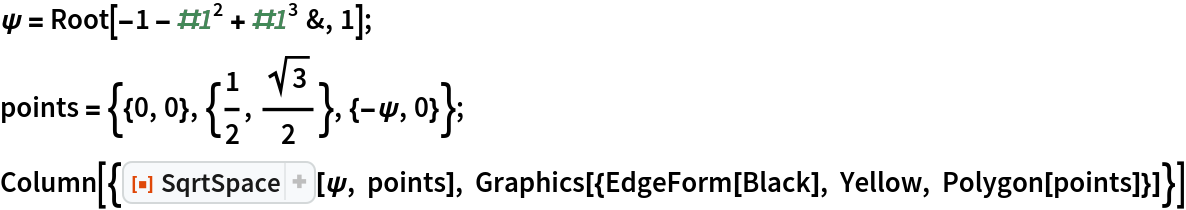Out=Convert back to the original points:

 In:=Out=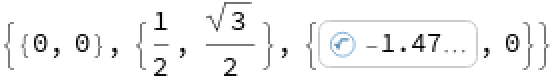### Properties and Relations (2)

Under "Neat Examples" in GeometricScene, there is a mysterious output after "Decompose a triangle into similar triangles":

 In:=Out=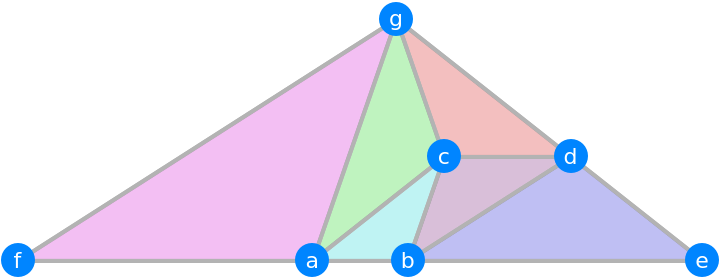This triangle is in the algebraic number field / geometric space of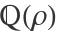where ρ is the plastic constant:

 In:=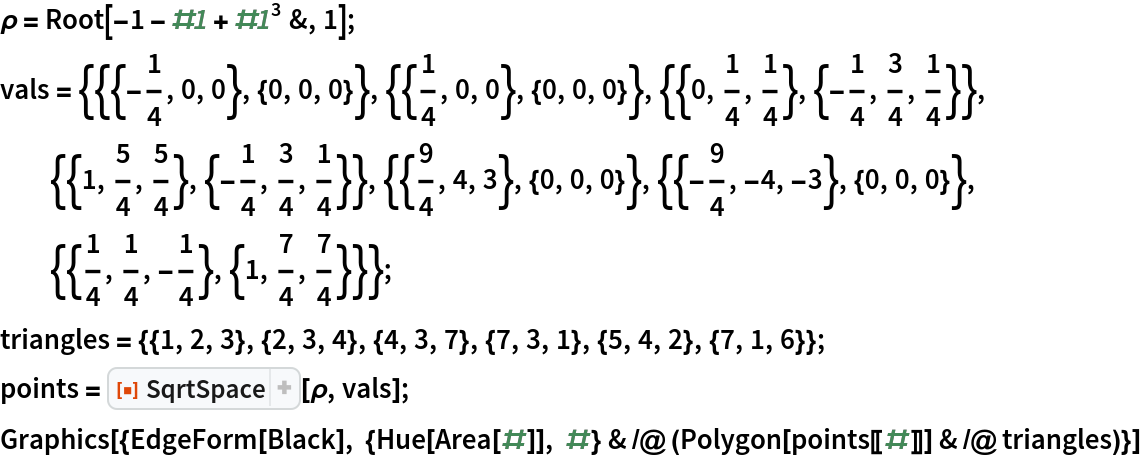Out=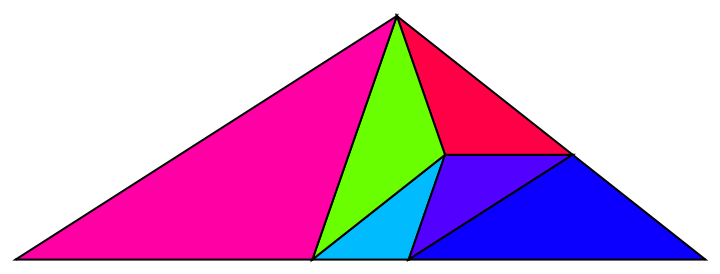Convert the points back to original values:

 In:=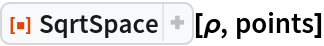Out=A simple application of ToNumberField does not recognize the points as being in eitheror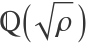, but does recognize the values when they get squared:

 In:=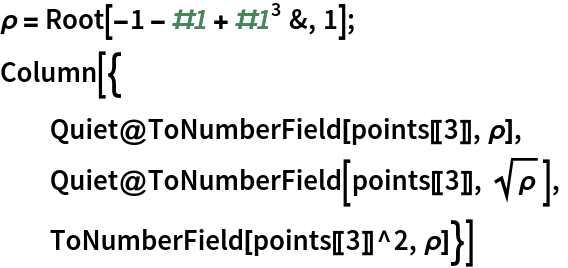Out=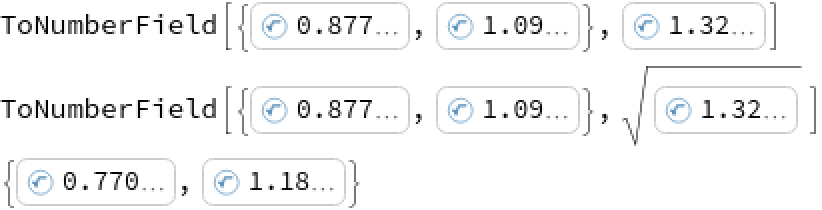These values are algebraic numbers:

 In:=Out=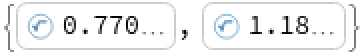The actual point is also a pair of algebraic numbers:

 In:=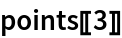Out=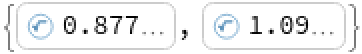The signs here happen to be positive, so taking the square root of the algebraic version does not require extra steps:

 In:=Out=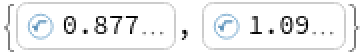### Neat Examples (3)

Convert 19 points from the algebraic number fieldof the plastic constant ρ into 3D coordinates:

 In:=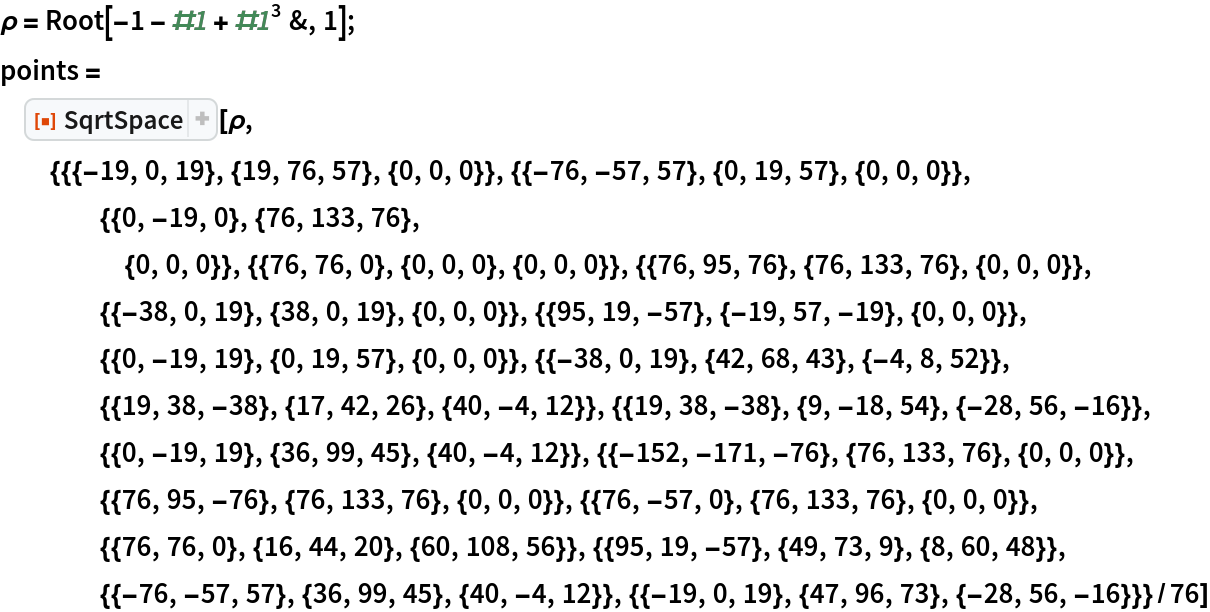Out=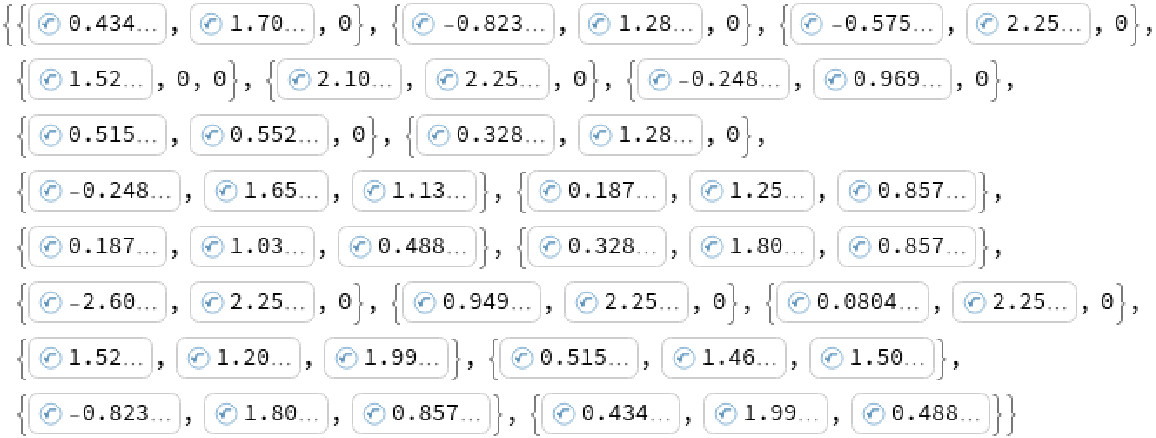Find the distances between these points in terms of powers of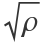:

 In:=Out=Plot out the points:

 In:=Out=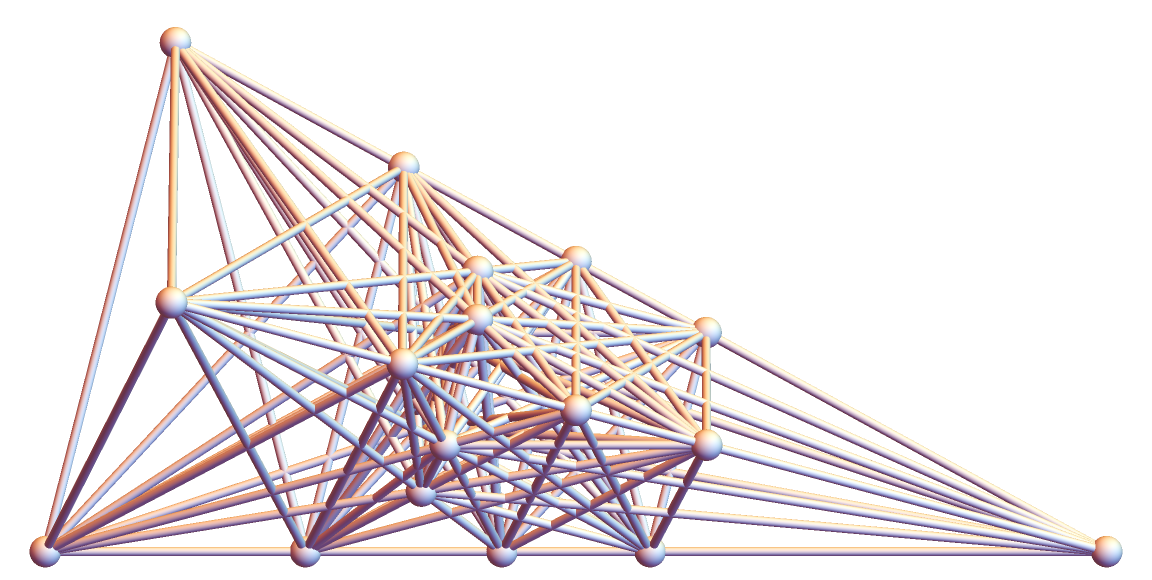## Requirements

Wolfram Language 11.3 (March 2018) or above

## Version History

• 1.0.0 – 27 February 2019